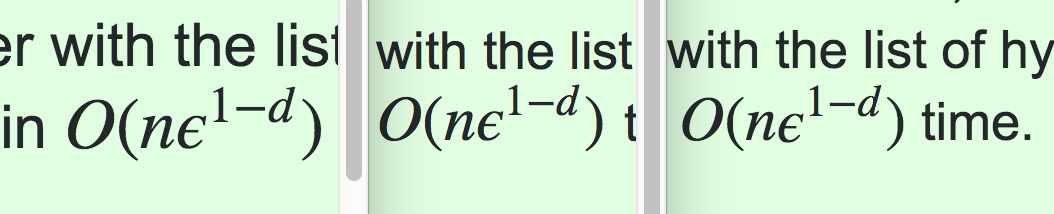LaTeX display of exponential pushed down with respect to baseline

This is hardly important, but ... In an answer, I used the LaTeX O(n \epsilon^{1-d}) to display $O(n \epsilon^{1-d})$, but this is what it looks like to me (in Chrome 64.0.3282.140 under MacOS 10.13.3):Note the $\epsilon^{1-d}$ is pushed downward, likely by the line above. But the use of the same LaTeX in this post looks fine (to me).

Is this a MathJax / Chrome bug?

• What MathJax renderer are you using? Does the display change if you switch to another one? Feb 11 '18 at 14:26
• @MartinSleziak: I don't know. How can I determine which MathJax renderer is installed? Feb 11 '18 at 14:39
• You can change the renderer in the MathJax menu which you get by right clicking on any MathJax formula. $x^2+y^2=z^2$ The links I gave in the previous comment and in this one show a few screenshots. Feb 11 '18 at 14:47
• @MartinSleziak: Thanks. I see I am using HTML-CSS. MathML is not supported natively in Chrome. Feb 11 '18 at 14:53